DAY 23
0
Modern Web

Day 23. slate × Operation × L-transform• Path.transform
• Point.transform
• Range.transform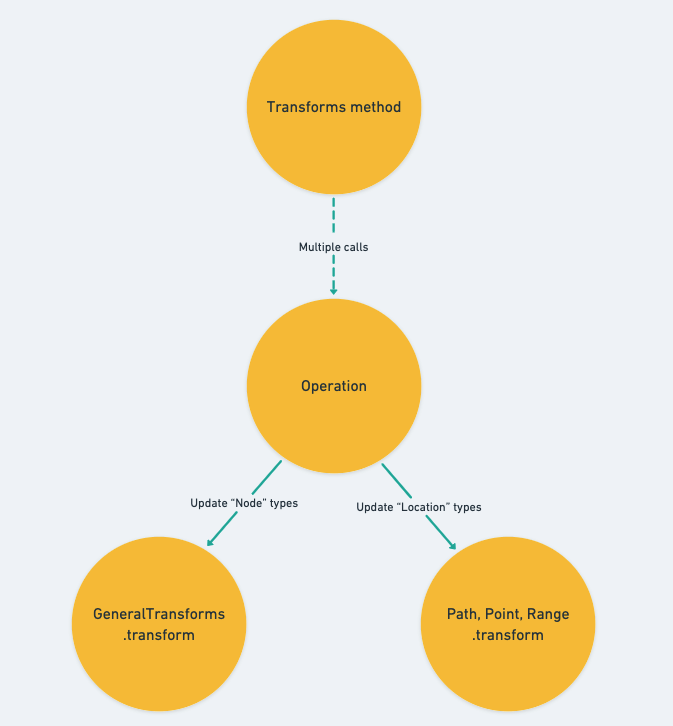Path.transform

• path ：主要傳入接受更新的路徑資料，會因應傳入的 operation 回傳相對應更新過後的 Immutable value 。
• operation ：執行的 Operation 資料，下方的內容會很常取出在 operation.path alias 為 op 使用，之後在這個小節看到 op 代表的就是 operation.path 的 value 。

/**
* Transform a path by an operation.
*/
transform(
path: Path,
operation: Operation,
options: { affinity?: 'forward' | 'backward' | null } = {}
): Path | null {
return produce(path, p => {
// ... Path.transform implementation
});
}

insert_node

1. 完全相同的路徑
2. 同層且位於 op 之後的 sibling 的路徑
3. op 為祖先之路徑

case 'insert_node': {
const { path: op } = operation

if (
Path.equals(op, p) ||
Path.endsBefore(op, p) ||
Path.isAncestor(op, p)
) {
p[op.length - 1] += 1
}
break
}
1. 容易懂，插入的節點位於同一層又在它的位置以前，所以要將自己的 index + 1。
1. 就稍嫌 tricky 一點了，注意到它指定 + 1 的陣列 index 是 op 的 index 值，所以如果今天插入的節點路徑是位於 path 上方的祖先時，我們會將與 op index 同層的值 + 1 ，因為是它祖先那一層被異動到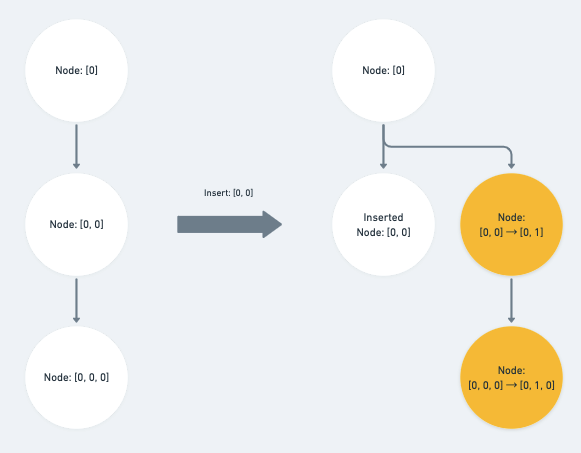remove_node

case 'remove_node': {
const { path: op } = operation

if (Path.equals(op, p) || Path.isAncestor(op, p)) {
return null
}

// ... else if statement

break
}

else if (Path.endsBefore(op, p)) {
p[op.length - 1] -= 1
}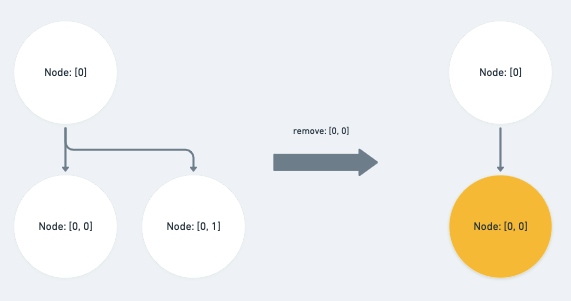merge_node

『合併節點』可以分為兩種情形：

• op 與 path 相等，或為 path 之前的 sibling ：

直接將 path 對應的 index 值 - 1

case 'merge_node': {
const { path: op, position } = operation

if (Path.equals(op, p) || Path.endsBefore(op, p)) {
p[op.length - 1] -= 1
}

// ... else if statement

break
}

• op 為 path 的祖先路徑：

除了將對應的 index 值 -1 之外，我們還需要子層的 index 值去加上 position ，也就是它前一個 sibling 的 index 值

else if (Path.isAncestor(op, p)) {
p[op.length - 1] -= 1
p[op.length] += position
}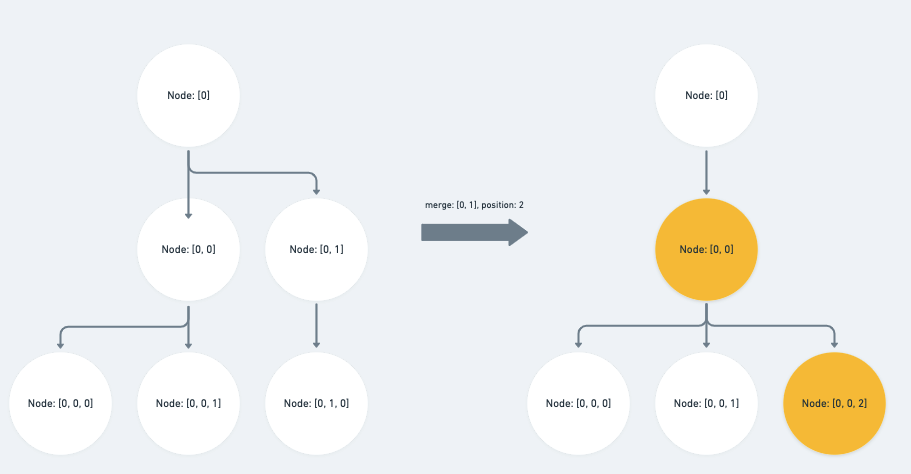split_node

『拆分節點』可以分為三種情形：

• oppath 相等

這裡就會受到 options.affinity 所影響了， affinity 在這裡代表的是將原始的 path 指向的節點向後（ forward ）或是向前（ backward ）做拆分，如果是向後拆分則將原始路徑的 index + 1 ，向前則不需要做任何操作。

case 'split_node': {
const { path: op, position } = operation

if (Path.equals(op, p)) {
if (affinity === 'forward') {
p[p.length - 1] += 1
} else if (affinity === 'backward') {
// Nothing, because it still refers to the right path.
} else {
return null
}
}

// ... other statements

break
}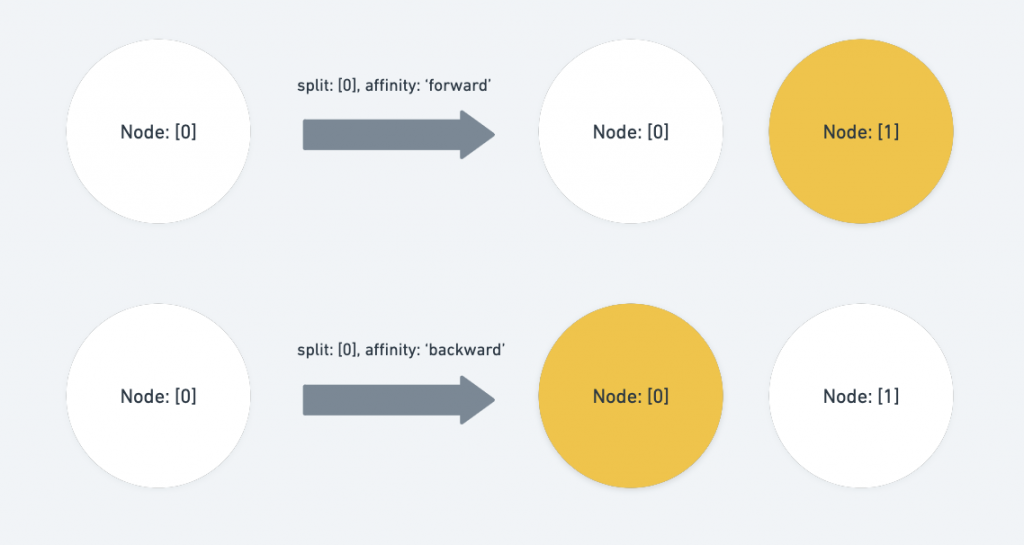• oppath 同層，並位於 path 前面的 sibling

直接將 path 的 index 值 + 1 。

else if (Path.endsBefore(op, p)) {
p[op.length - 1] += 1
}

• oppath 的祖先，且 op index 的子層對應到的 path index 位於 position 的後方：

同層的 index 值 + 1 ，同時子層節點扣除掉拆分的 position offset

else if (Path.isAncestor(op, p) && path[op.length] >= position) {
p[op.length - 1] += 1
p[op.length] -= position
}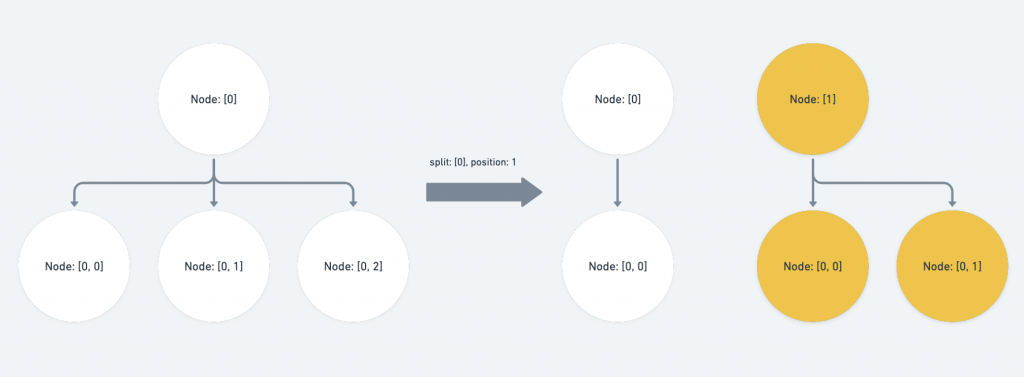move_node

case 'move_node': {
const { path: op, newPath: onp } = operation

// If the old and new path are the same, it's a no-op.
if (Path.equals(op, onp)) {
return
}

// ... If else implementations

break
}

• op （ Operation 裡欲移動的舊路徑 ）為 p 的祖先或 opp 的路徑相等：

此時的 p 被包含在 Operation 的搬遷範圍內，我們要將 onp （ Operation 裡欲移動到的新路徑 ）補上 op index 之後、 p 所涵蓋到的子層。

if (Path.isAncestor(op, p) || Path.equals(op, p)) {
const copy = onp.slice()

// ... if statement for the edge case

return copy.concat(p.slice(op.length))
}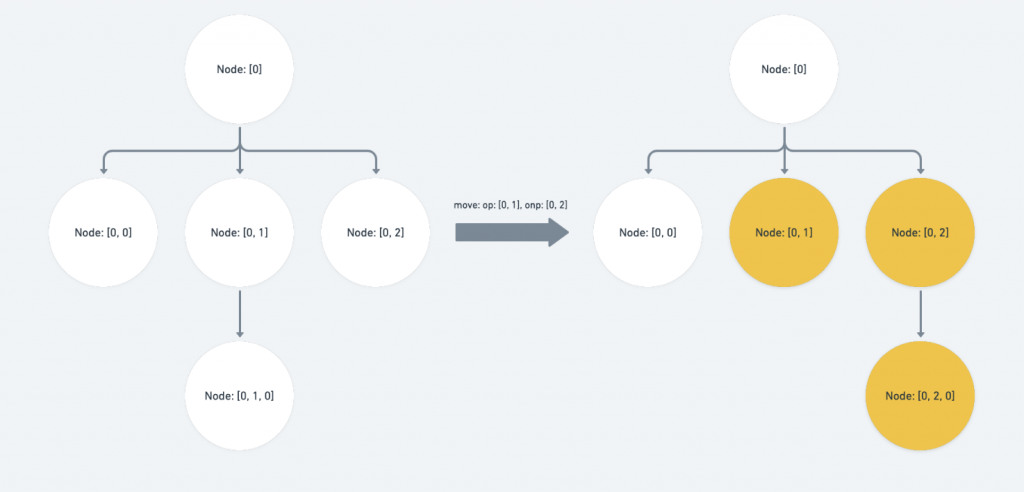上圖的結果就是：[0, 2] 會向前調動到 [0, 1] ，而 [0, 1] 的整個 branch 則會一起向後移動一個 sibling 。

再來這個情形還有一個 edge case 是需要注意的，也就是當 op 位於 onp 之前，同時為 onp 的上層路徑。

此時我們會需要調整 onpop 層的 index value 將它 -1 ，因為 op 會遭到移除

if (Path.endsBefore(op, onp) && op.length < onp.length) {
copy[op.length - 1] -= 1
}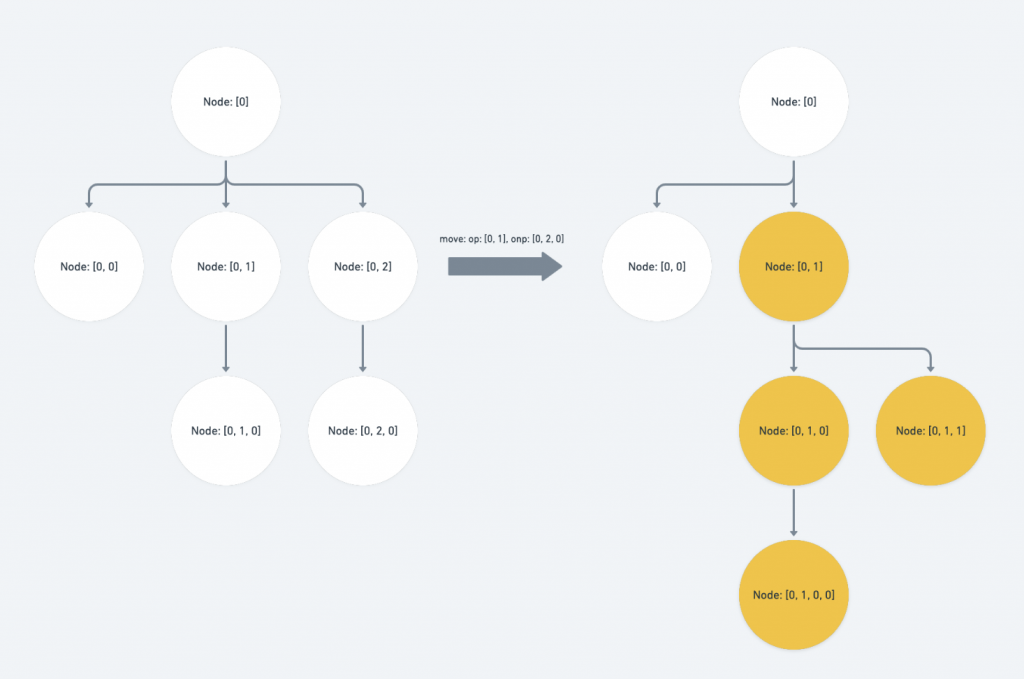• oponp 同層，且 onpp 的祖先或 onpp 的路徑相等：

此時我們需要調整 pop index 層的 value 。如果 op 位於 p 之前，代表移動後 value 會因為該層前面的 sibling 遭到拔除因而需要 -1 ；反之則代表該層會有新的 sibling 被移動到前面因而需要 +1

else if (
Path.isSibling(op, onp) &&
(Path.isAncestor(onp, p) || Path.equals(onp, p))
) {
if (Path.endsBefore(op, p)) {
p[op.length - 1] -= 1
} else {
p[op.length - 1] += 1
}
}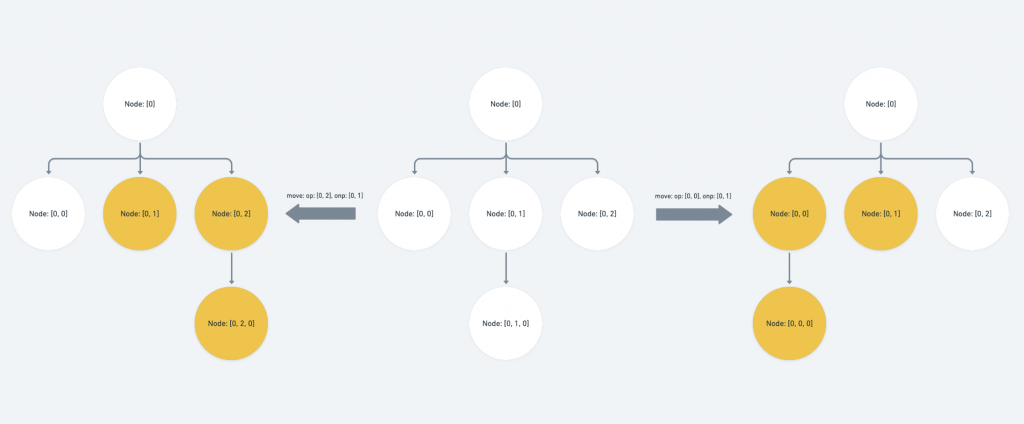• onp 位於 p 之前，或 onpp 相等，或 onpp 之祖先：

代表我們要將某一組路徑搬遷到 p 之前，因此我們需要將 ponp index 層的 value +1

else if (
Path.endsBefore(onp, p) ||
Path.equals(onp, p) ||
Path.isAncestor(onp, p)
) {
// ... if statement for the edge case

p[onp.length - 1] += 1
}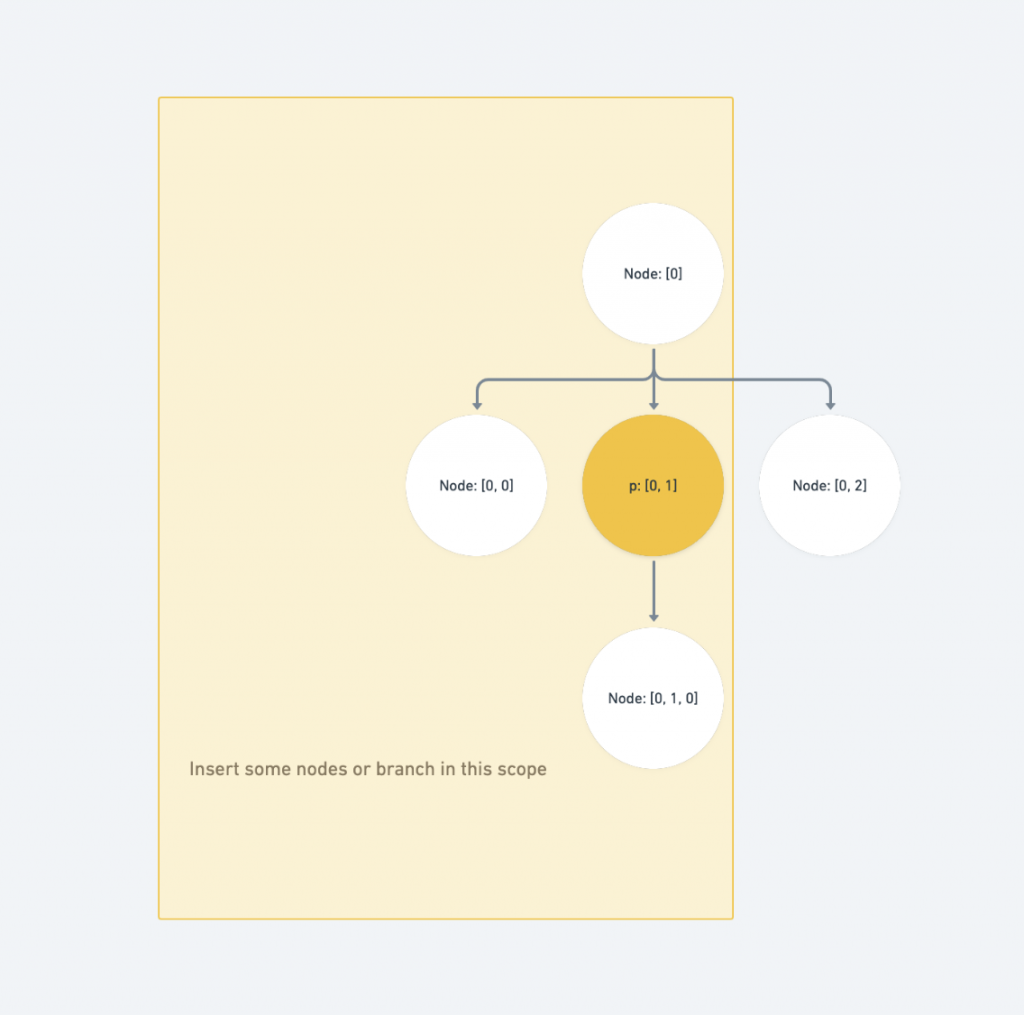需要注意的 edge case 為：如果要搬遷的 op 位於 p 之前，代表會有一組路徑從 p 之前的位置受到搬遷，我們需要扣除 pop 層的 index value

if (Path.endsBefore(op, p)) {
p[op.length - 1] -= 1
}

• op 位於 p 之前：

代表我們要將某一組路徑從 p 之前搬遷走，因此我們需要將 pop index 層的 value -1

else if (Path.endsBefore(op, p)) {
if (Path.equals(onp, p)) {
p[onp.length - 1] += 1
}

p[op.length - 1] -= 1
}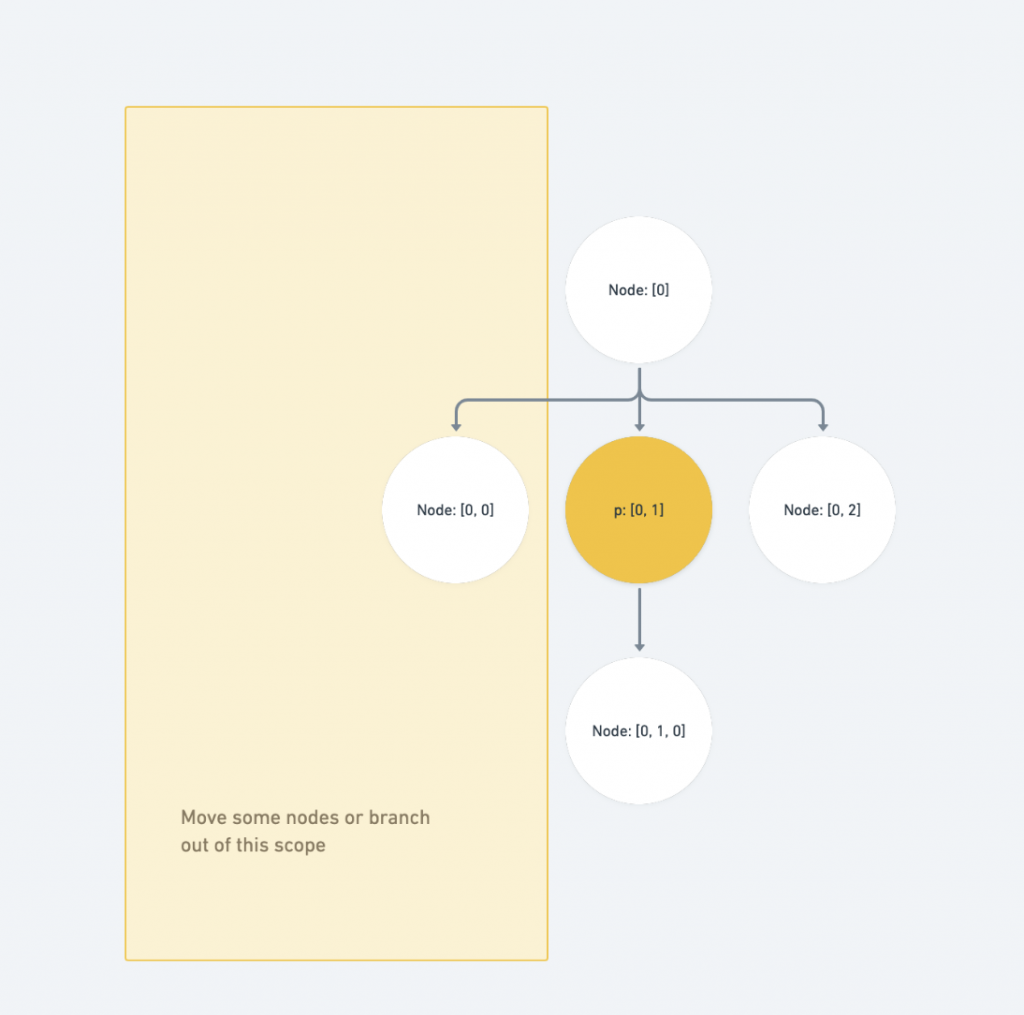Point.transform

• point ：主要傳入接受更新的 Point 資料，會因應傳入的 operation 回傳相對應更新過後的 Immutable value 。
• op ：執行的 Operation 資料 。

/**
* Transform a point by an operation.
*/
transform(
point: Point,
op: Operation,
options: { affinity?: 'forward' | 'backward' | null } = {}
): Point | null {
return produce(point, p => {
const { affinity = 'forward' } = options
const { path, offset } = p
// ... Point.transform implementation
});
}

insert_node & move_node

case 'insert_node':
case 'move_node': {
p.path = Path.transform(path, op, options)!
break
}

insert_text

case 'insert_text': {
if (Path.equals(op.path, path) && o <= offset) {
p.offset += op.text.length
}

break
}

merge_node

op.pathpath 為同一個路徑時，將 position 加進 offset 裡，再將 path 丟入 Path.transform

case 'merge_node': {
if (Path.equals(op.path, path)) {
p.offset += op.position
}

p.path = Path.transform(path, op, options)!
break
}

remove_text

case 'remove_text': {
if (Path.equals(op.path, path) && op.offset <= offset) {
p.offset -= Math.min(offset - op.offset, op.text.length)
}

break
}

remove_node

case 'remove_text': {
if (Path.equals(op.path, path) && op.offset <= offset) {
p.offset -= Math.min(offset - op.offset, op.text.length)
}

break
}

split_node

case 'split_node': {
if (Path.equals(op.path, path)) {
// Implementation
} else {
p.path = Path.transform(path, op, options)!
}

break
}
}

if (op.position === offset && affinity == null) {
return null
} else if (
op.position < offset ||
(op.position === offset && affinity === 'forward')
) {
p.offset -= op.position

p.path = Path.transform(path, op, {
...options,
affinity: 'forward',
})!
}

Range.transform

/**
* Transform a range by an operation.
*/

transform(
range: Range,
op: Operation,
options: {
affinity?: 'forward' | 'backward' | 'outward' | 'inward' | null
} = {}
): Range | null {
// ... affinity statement control

return produce(range, r => {
const anchor = Point.transform(r.anchor, op, { affinity: affinityAnchor })
const focus = Point.transform(r.focus, op, { affinity: affinityFocus })

if (!anchor || !focus) {
return null
}

r.anchor = anchor
r.focus = focus
})
},

const { affinity = 'inward' } = options
let affinityAnchor: 'forward' | 'backward' | null
let affinityFocus: 'forward' | 'backward' | null

if (affinity === 'inward') {
if (Range.isForward(range)) {
affinityAnchor = 'forward'
affinityFocus = 'backward'
} else {
affinityAnchor = 'backward'
affinityFocus = 'forward'
}
} else if (affinity === 'outward') {
if (Range.isForward(range)) {
affinityAnchor = 'backward'
affinityFocus = 'forward'
} else {
affinityAnchor = 'forward'
affinityFocus = 'backward'
}
} else {
affinityAnchor = affinity
affinityFocus = affinity
}

Operation 章節到這邊總算告一個段落了，這個章節的後兩篇我們非常深入地去探討了 Operation 底層的程式碼是如何運作的，希望這能讓讀者在以 slate 為基礎開發編輯器時能更清楚在每一個操作的背後，編輯器實際上都是如何更新的。

不要像筆者一開始一樣純粹靠對 api 名稱的直覺做開發ＸＤ

Normalization 在 slate 中也是一個非常重要的功能，它也支援開發者加入自定義的 Normalizing constraints 。